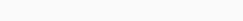# Solved – Sample from a Normal-Inverse-Chi-Squared distribution

Is there a way to draw samples from the following distribution in Python?Unfortunately I wasn't able to find helpful information in the scipy help.

Contents

According to this document, on page 2, if \$Z sim\$ Normal Inverse \$chi^2\$ distribution\$(m,k,r,s)\$, then \$Z = (W,V)\$ such that \$\$dfrac{rs}{V} sim chi^2_r quad text{and} quad W|(V = v) sim Nleft(m, vk^{-1} right).\$\$

Thus to sample from a Normal-Inverse \$chi^2\$ distribution, you sample \$V\$ first and then sample \$W\$. For your parameters you have, \$Z sim\$ N-Inv-\$chi^2\$\$(beta, sigma^2Omega^{-1}; v_0, s_0^2).\$

Thus, you first sample \$V = v\$ such that, \$\$dfrac{v_0 s_0^2}{V} sim chi^2_{v_0}. \$\$

This can be done by first sample an \$x\$ from \$chi^2_{v_0}\$ and then set \$v = v_0s_0^2/x\$.

Next, using this \$v\$, you want to sample \$W = w\$ such that, \$\$W|(V = v) sim N(beta_0, vsigma^{-2}Omega). \$\$

Thus the draw \$(w,v)\$ will be a draw from the N-Inv \$chi^2\$.

(I am not familiar with Python, but I am sure there are functions that will help you draw from a \$chi^2\$ and Normal distributions.)

Rate this post